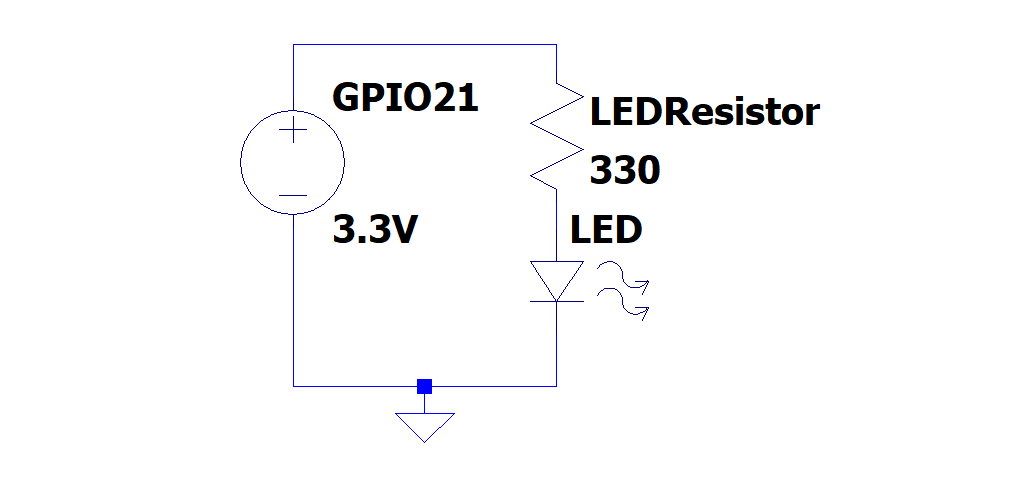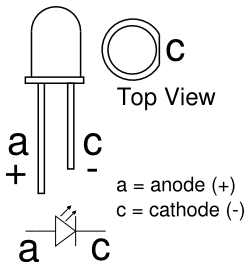# LEDs

Created: General information on using LEDs in electric circuits

Updated: 03 September 2023

LED is short for Light Emitting Diode

# Symbol

LED’s are represented with the Diode symbol with 2 arrows added:# Connecting

When connecting an LED in a circuit it must be connected:

1. in series with a resistor
2. in the correct direction

Below is an example circuit:## Series Resistor

The resistor to be connected with a specific LED can be calculated by:

$$R_{LED} = \frac{V_{source} - V_{LED}}{I_{LED}}$$

Where:

• $V_{source}$ is the voltage over the LED and Resistor
• $V_{LED}$ is the LED’s required voltage
• $I_{LED}$ is the current required across the LED

## Direction

The direction that an LED is connected in is very important, both for functioning purposes as well as to ensure the LED is not damaged.

Below is a diagram showing the direction for LED connection, from Starting Electronics